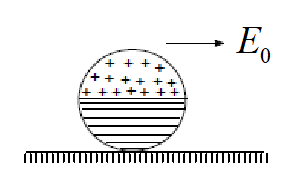A disc having uniform surface charge density +σ  in upper half and −σ in lower half is placed on a rough horizontal surface as shown in figure. A uniform electric field is set up as shown. If mass of disc is M and its radius is R, as well sufficient friction is present to prevent slipping, the acceleration of disc is

# A disc having uniform surface charge density $+\sigma$  in upper half and $-\sigma$ in lower half is placed on a rough horizontal surface as shown in figure. A uniform electric field is set up as shown. If mass of disc is M and its radius is R, as well sufficient friction is present to prevent slipping, the acceleration of disc is1. A

$\frac{8\sigma {R}^{2}{E}_{0}}{9\text{\hspace{0.17em}\hspace{0.17em}\hspace{0.17em}\hspace{0.17em}\hspace{0.17em}}M}$

2. B

$\frac{9\sigma {R}^{2}{E}_{0}}{8\text{\hspace{0.17em}\hspace{0.17em}\hspace{0.17em}\hspace{0.17em}\hspace{0.17em}}M}$

3. C

$\frac{4\sigma {R}^{2}{E}_{0}}{3\text{\hspace{0.17em}\hspace{0.17em}\hspace{0.17em}\hspace{0.17em}\hspace{0.17em}}M}$

4. D

$\frac{3\sigma {R}^{2}{E}_{0}}{4\text{\hspace{0.17em}\hspace{0.17em}\hspace{0.17em}\hspace{0.17em}\hspace{0.17em}}M}$

Register to Get Free Mock Test and Study Material

+91

Verify OTP Code (required)

### Solution:

[Step 1]: Dipole moment

[Step 2]:  $P=\frac{4{R}^{3}\sigma }{3}$
[Step 3]:  $f=Ma$
[Step 4]:

[Step 5]:
[Step 6]: For pure rolling  $a=\frac{8}{9}\frac{\sigma {E}_{0}{R}^{2}}{M}$Register to Get Free Mock Test and Study Material

+91

Verify OTP Code (required)